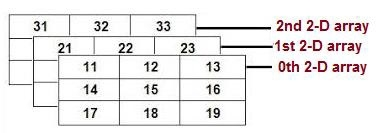# Multidimensional Arrays in C / C++

In C/C++, Multidimensional array is defined in simple words as array of arrays. In multidimensional arrays data are stored in tabular form (in row major order). The following diagram shows the memory allocation strategy for a multidimensional array with dimension 3 x 3 x 3.## Algorithm

Begin
Declare dimension of the array.
Dynamic allocate 2D array a[][] using new.
Fill the array with the elements.
Print the array.
Clear the memory by deleting it.
End

## Example Code

Live Demo

#include <iostream>
using namespace std;
int main() {
int B = 4;
int A = 5;
int** a = new int*[B];
for(int i = 0; i < B; ++i)
a[i] = new int[A];
for(int i = 0; i < B; ++i)
for(int j = 0; j < A; ++j)
a[i][j] = i;
for(int i = 0; i < B; ++i)
for(int j = 0; j < A; ++j)
cout << a[i][j] << "\n";
for(int i = 0; i < A; ++i)
delete [] a[i];
delete [] a;
return 0;
}

## Output

0
0
0
0
0
1
1
1
1
1
2
2
2
2
2
3
3
3
3
3